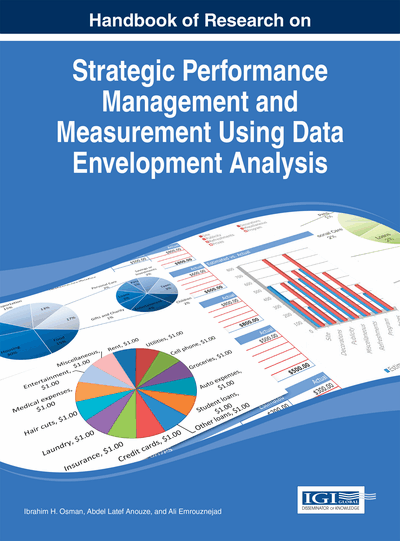# Introduction to Data Envelopment Analysis and its Applications

DOI: 10.4018/978-1-4666-4474-8.ch004
OnDemand:
(Individual Chapters)
Available
\$37.50
No Current Special Offers

## Abstract

This chapter provides the theoretical foundation and background on Data Envelopment Analysis (DEA) method and some variants of basic DEA models and applications to various sectors. Some illustrative examples, helpful resources on DEA, including DEA software package, are also presented in this chapter. DEA is useful for measuring relative efficiency for variety of institutions and has its own merits and limitations. This chapter concludes that DEA results should be interpreted with much caution to avoid giving wrong signals and providing inappropriate recommendations.
Chapter Preview
Top

## Introduction

The theoretical foundation of the Data Envelopment Analysis (DEA) can be traced from economic and production theories. Production is defined as a process of transforming inputs such as land, labor, and capital or valuable resources into goods and services. The underlying issue of the efficiency of combining inputs to produce some outputs can be measured by efficiency measures. Economic efficiency is achieved where production costs are lower and consumers demand the combination of goods and services to be produced. Technical efficiency and allocative efficiency are the two components of economic efficiency measure. Technical efficiency refers to the waste avoidance, either by producing much output as technology and input usage needed or by using as little input required by technology and output production (Fried et al., 2008). The analysis of technical efficiency can either have an output orientation or an input orientation. The allocative efficiency refers to the combination of inputs to produce a given quantity of outputs given the prevailing input prices (Coelli et al., 2005).

The Cobb-Douglas model is a commonly used production function and expressed in this functional form: for Q as an output, and two inputs: labor L and capital K. The non-linear equation form of this function is Q = ALaKb . A is a constant that depends on the units of measurement of output Q, and labor L and capital by K. The coefficients a and b are the elasticity of outputs with respect to labor and capital inputs, respectively. Furthermore, a and b can measure returns to scale. If a + b = 1, then output remains the same, and efficiency indicates constant returns to scale (CRS). If a + b <1, then output is lesser than input and signifies decreasing returns to scale (DRS). If a + b >1, then output is greater than input and indicates increasing returns to scale (IRS). The production concept of returns to scale is also considered in calculating productive efficiency of a firm using the data envelopment analysis approach. It relates to increasing and decreasing efficiency based on firm’s sizes. When two extremes (IRS and DRS) are combined, it will necessitate variable returns to scale (VRS). As such, VRS is defined as the ability of the firm to catch up, given limitations such as constraints on finances, market imperfection, firm’s sizes, etc. that may cause the firm not to be operating at optimal scale. On the other hand, the constant returns to scale (CRS) signifies that the firm is able to scale the inputs and outputs linearly without increasing or decreasing efficiency (Ramanathan, 2003).

Productivity is defined as the ratio of outputs to its inputs. When a firm uses a single input to produce a single output, the ratio becomes a trivial calculation. In some cases, a firm uses several inputs to produce several outputs, then we can use the method for aggregating inputs and or outputs to obtain a ratio measure of productivity measures (Coelli et al., 2005:3). The difference between output growth and input growth is the productivity growth, in which the aggregation requirement is also applied (Fried et al., 2008).

In the production, the decision-making unit (DMU) is the firm. The selected variables are quantities of outputs to be produced from the quantities of inputs allowed. The input-output mix selected by a DMU must be technically feasible to produce the desirable output bundle selected from the associated input bundle.

Koopmans (1951) provided a more formal definition of technical efficiency: A firm is only technically efficient if it operates on the frontier and that all associated slacks are zero (Coelli, 2005, 164). However, a popular measure of microeconomic efficiency measurement began from the early works of Debreu (1951) and Farrel (1957), which defined a simple measure of firm’s production efficiency that could deal with multiple inputs, not at a time, but over a period of time. In an input-saving orientation, their measure is defined as the maximum equiproportionate or radial reduction in inputs with given technology and outputs. In an output-increasing orientation, their measure is defined as the maximum radial expansion in outputs with given technology and inputs. In both input and output orientations, a value of unity of one indicates technical efficiency because no radial adjustment is feasible, while a value different from a unity of one indicates technical inefficiency (Fried et al., 2008). Thereafter, the Debreu-Farell measures were applied increasingly in the 1990s for measuring production efficiency.

## Complete Chapter List

Search this Book:
Reset